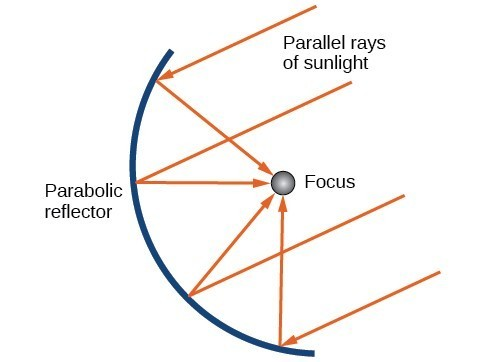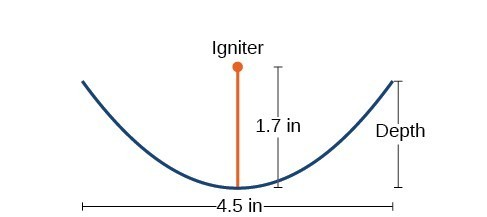## Solving Applied Problems Involving Parabolas

As we mentioned at the beginning of the section, parabolas are used to design many objects we use every day, such as telescopes, suspension bridges, microphones, and radar equipment. Parabolic mirrors, such as the one used to light the Olympic torch, have a very unique reflecting property. When rays of light parallel to the parabola’s axis of symmetry are directed toward any surface of the mirror, the light is reflected directly to the focus. This is why the Olympic torch is ignited when it is held at the focus of the parabolic mirror.Figure 12. Reflecting property of parabolas

Parabolic mirrors have the ability to focus the sun’s energy to a single point, raising the temperature hundreds of degrees in a matter of seconds. Thus, parabolic mirrors are featured in many low-cost, energy efficient solar products, such as solar cookers, solar heaters, and even travel-sized fire starters.

### Example 7: Solving Applied Problems Involving Parabolas

A cross-section of a design for a travel-sized solar fire starter is shown in Figure 13. The sun’s rays reflect off the parabolic mirror toward an object attached to the igniter. Because the igniter is located at the focus of the parabola, the reflected rays cause the object to burn in just seconds.

1. Find the equation of the parabola that models the fire starter. Assume that the vertex of the parabolic mirror is the origin of the coordinate plane.
2. Use the equation found in part (a) to find the depth of the fire starter.Figure 13. Cross-section of a travel-sized solar fire starter

### Solution

1. The vertex of the dish is the origin of the coordinate plane, so the parabola will take the standard form ${x}^{2}=4py$, where $p>0$. The igniter, which is the focus, is 1.7 inches above the vertex of the dish. Thus we have $p=1.7$.
$\begin{array}{ll}{x}^{2}=4py\hfill & \begin{array}{cccc}& & & \end{array}\text{Standard form of upward-facing parabola with vertex (0,0)}\hfill \\ {x}^{2}=4\left(1.7\right)y\hfill & \begin{array}{cccc}& & & \end{array}\text{Substitute 1}\text{.7 for }p.\hfill \\ {x}^{2}=6.8y\hfill & \begin{array}{cccc}& & & \end{array}\text{Multiply}.\hfill \end{array}$
2. The dish extends $\frac{4.5}{2}=2.25$ inches on either side of the origin. We can substitute 2.25 for $x$ in the equation from part (a) to find the depth of the dish.
$\begin{array}{ll}\text{ }{x}^{2}=6.8y\hfill & \text{Equation found in part (a)}.\hfill \\ {\left(2.25\right)}^{2}=6.8y\hfill & \text{Substitute 2}\text{.25 for }x.\hfill \\ \text{ }y\approx 0.74 \hfill & \text{Solve for }y.\hfill \end{array}$

The dish is about 0.74 inches deep.

### Try It 7

Balcony-sized solar cookers have been designed for families living in India. The top of a dish has a diameter of 1600 mm. The sun’s rays reflect off the parabolic mirror toward the “cooker,” which is placed 320 mm from the base.

a. Find an equation that models a cross-section of the solar cooker. Assume that the vertex of the parabolic mirror is the origin of the coordinate plane, and that the parabola opens to the right (i.e., has the x-axis as its axis of symmetry).

b. Use the equation found in part (a) to find the depth of the cooker.

Solution